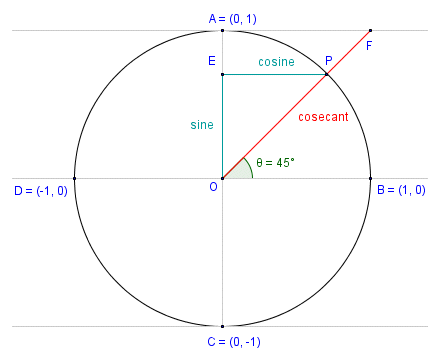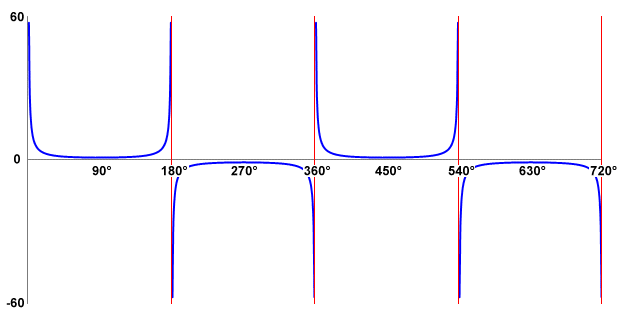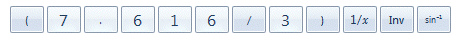# The Cosecant Function

The cosecant function is a trigonometric function, one of three reciprocal functions that we look at in these pages, the other two being the secant function and the cotangent function. A reciprocal function is one that is the reciprocal (or multiplicative inverse) of another function (see below). The cosecant function (usually abbreviated as csc) is the reciprocal function of the sine function. As with the other trigonometric functions in modern use, the cosecant function was certainly known to Arab scholars, and tables of cosecant values are known to have been in existence by the tenth century CE. In order to clarify exactly how the cosecant function and the sine function are related, consider the diagram below.The cosecant as a line segment of the unit circle

Like the other trigonometric functions, the cosecant can be represented as a line segment associated with the unit circle. The diagram shows the cosecant for an angle of rotation θ of forty-five degrees (measured anti-clockwise from the positive x-axis). Line segment AF lies on the line that is tangent to the circle at point A. Line segment OF (shown in red) represents the cosecant, and is an extension of line segment OP. Remember that point P is a point on the circumference of the circle whose x and y coordinates represent the value of cos (θ ) and sin (θ ) respectively (the line segments representing the sine and cosine are also shown). Click here to see an interactive demonstration that uses the unit circle to show how the sine and the cosecant functions relate to one another (note: your browser must support Java in order for the interactive page to work). If you haven't already deduced the fact from the diagram, you will see from the interactive demo that as the value of sin (θ ) approaches one, so does the value of csc (θ ). As the value of sin (θ ) approaches zero, however, the value of csc (θ ) tends to infinity. The value of csc (θ ) when sin (θ ) equals zero is thus said to be undefined.

Obviously, since the cosecant function is the reciprocal of the sine function, it can be expressed in terms of the sine function as:

 csc (θ )  = 1 sin (θ )

Since the sine is represented by the y coordinate of point P (i.e. the point at which the terminal side of angle θ intersects the circumference of the unit circle), we can also conclude that:

 csc (θ )  = 1 y

We can also define the cosecant in terms of a right-angled triangle. If you have read the pages in this section covering the three main trigonometric functions (sine, cosine and tangent) you will by now be familiar with the diagram below.In triangle ABC, csc (θ )  =  c/a

In right-angled triangle ABC, the sine of angle θ is the quotient of the opposite and the hypotenuse (i.e. the sides labelled a and c respectively). Since the cosecant function is the reciprocal of the sine function, then the cosecant of angle θ will be the quotient of the hypotenuse and the opposite. This gives us:

 csc (θ )  = 1 = hypotenuse = c sin (θ ) opposite a

For those interested in such things, we have used Microsoft Excel to produce our own table of cosecant values for angles ranging from zero degrees () up to three hundred and sixty degrees (360°) in increments of one tenth of a degree. To see the table, click here.

If you study the table, you can see that there is a significant range of angles centred around ninety degrees for which the value returned by the cosecant function is quite small and changes relatively slowly. For angles close to either zero degrees () or one hundred and eighty degrees (180°) however, the value returned by the cosecant function is much bigger. This is to be expected, since the value of sin (θ ) will be very small for these angles, so its reciprocal will be correspondingly large. In fact, the value returned by the cosecant function for an angle of either zero degrees or one hundred and eighty degrees is considered to be undefined, since the equation csc (θ ) = 1/sin (θ ) will involve division by zero. The same is true for an angle of three hundred and sixty degrees (360°). In fact, it will apply to any angle for which the sine value is zero. We present below the graph of the cosecant function for angles in the range zero degrees to seven hundred and twenty degrees (720°).A graph of the cosecant function for angles in the range to 720°

Because the absolute value of the sine of an angle will never exceed one, the reciprocal of the sine of an angle will never be less than one. So, just as the cosecant value is undefined for any angle for which the sine is zero, it will always be one for any angle for which the sine is one, and minus one (-1) for any angle for which the sine is minus one. In fact, as you can see from both the table of cosecant values and the graph of the cosecant function, the cosecant value is negative for all angles for which the sine is negative. Like the graph of the tangent function, the graph of the cosecant function can include some very large positive and negative peaks, as the value of sin (θ ) tends to zero. Unlike the tangent function however, the period of the cosecant function is three hundred and sixty degrees (360°), or . Like the sine function, the cosecant function returns positive values for angles in Quadrants I and II, and negative values for angles in Quadrants III and IV.

If you have read the page entitled "The Tangent Function" (or possibly even if you haven't) you will know that the vertical red lines on the graph are asymptotes. The asymptote of a curve is a line such that, as the distance between the curve and the line approaches zero, the value of the curve tends to infinity. In this case, the asymptotes can be considered to be the "gaps" in the graph where the value of csc (θ ) is undefined. Notice also that as the graph crosses the asymptote, the value of csc (θ ) transitions from its maximum positive value to its maximum negative value (or vice versa). You can also see how the values differ on either side of the asymptote by studying the table of cosecant values.

As with the other trigonometric functions we have looked at, we don't need to use tables to find the cosecant of an angle (or the angle corresponding to a given cosecant value), since any modern scientific calculator can do it for us, even though most calculators don't have a dedicated button for the cosecant function. Finding the cosecant of an angle with the built-in calculator provided in Microsoft Windows is still relatively straightforward. Let's assume we want to find the cosecant of an angle of eighty-five degrees (85°). You can find the Microsoft Windows built-in calculator by clicking on the start button in Windows 7 and selecting All Programs>Accessories>Calculator (other versions of Windows might require a different sequence of keystrokes). The scientific version of the calculator can be selected from the application's View menu. To find the cosecant of eighty-five degrees using the Windows calculator, enter the following keystrokes (if you don't have the Windows calculator, use any available calculator that can perform trig functions):Enter these keystrokes to find the cosecant of 85°

Since the cosecant function is the reciprocal of the sine function, all we have done here is to use the sine function button on the calculator, and then apply the calculator's built-in reciprocal function button (usually labelled 1/x) to the result. Make sure, by the way, that you have the calculator set to the Degrees mode (unless you are planning to enter a value for the angle in radians or gradians). If you have entered the keystrokes correctly, you should now see the following display:The calculator displays the value of csc(85°)

So far, so good. Now let's assume that you want to find the size of an acute angle in a right-angled triangle by first finding the quotient of the hypotenuse and opposite (i.e. the angle's cosecant). Providing you have values for the lengths of the sides of the triangle, you can find the value of the angle's cosecant easily enough. Finding the angle is not quite as straightforward as for the three main trigonometric functions, as most calculators do not have a dedicated button for the arccosecant function (which is the inverse of the cosecant function). Fortunately, there is a relatively simple way around that. Let's find the value of angle θ for the right-angled triangle illustrated below.We want to find the size of angle θ

For angle θ, sides c and a are the hypotenuse and the opposite respectively. We can actually find the size of the angle in a single operation on the Windows calculator. We do this by using brackets in the key sequence entered, in order to get the calculator to find the inverse of the quotient of the hypotenuse and opposite (i.e. the cosecant of the angle) first. We then apply the arcsine function to the result. Here is the key sequence to use:Enter these keystrokes to find the size of angle θ

You may have already worked out that what we did here was to find the sine of the angle by taking the reciprocal of the cosecant. We then simply applied the arcsine function to the result. We could of course simply reverse the order in which we enter the values (i.e. opposite / hypotenuse), in which case we don't need to use the reciprocal button. What should start to emerge for you at this point is the fact that all of the trigonometric functions are very closely interrelated, and that we can often replace an expression involving one trigonometric function with an equivalent expression involving a different trigonometric function. This can sometimes be very useful. If you have entered the keystrokes correctly, you should now see the following display:The calculator displays the value of angle θ

The cosecant function, in common with other trigonometric functions, can be represented as an infinite series. As we have mentioned elsewhere, the value returned by a trigonometric function for a given angle cannot be calculated using a simple algebraic formula. For most angles, an exact value can only be obtained as the sum of an infinite number of terms, which is obviously impossible to achieve. A reasonably accurate approximation of the value is found using the sum of a limited number of terms from the function's infinite series. The greater the number of terms included, the greater the accuracy of the result. The number of terms used will depend on the accuracy required, and on the computational resources available. The infinite series frequently cited for the cosecant function looks like this:

 csc (x)  =  x-1  + 1 x  + 7 x3  + 31 x5  +  · · · · 6 360 15,120